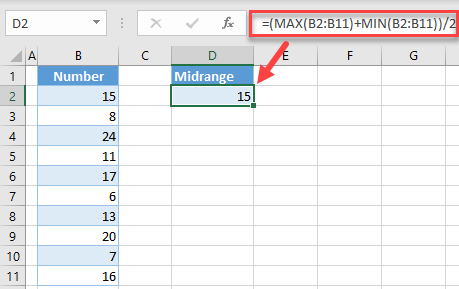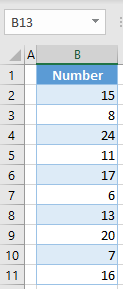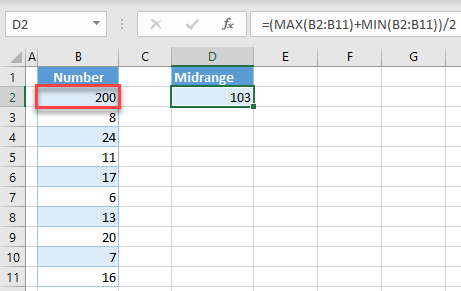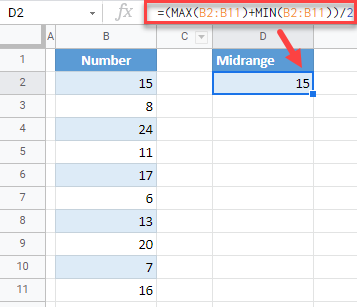# Find Midrange – Excel & Google Sheets

This tutorial demonstrates how to find the midrange of a dataset in Excel and Google Sheets.## What is Midrange?

The Midrange of a data set presents a center value of all values in a given dataset. It is actually an average of the largest and smallest value in a dataset.

## Calculate Midrange in Excel

We will calculate the midrange of this dataset:To achieve this, combine the MAX Function and MIN Function to find the largest and smallest value and then calculate their average:

``=(MAX(B2:B11) + MIN(B2:B11))/2``As a result, you have the midrange in cell D2. The largest value in the dataset is 24 and the smallest is 6, therefore the midrange is 15.

## Problems With Midrange

The possible issue while calculating a midrange is the case when you have one value that is by far larger or smaller than others. In the following dataset, the first value is 200.Because of that, the midrange is 103, which is not the real center of the dataset. In this case, it might be better to calculate the average (mean) or to calculate the median of the data set.

## Calculate Midrange in Google Sheets

Similar to Excel, you can also get a midrange in Google Sheets. To do this, in cell D2, enter the formula:

``=(MAX(B2:B11) + MIN(B2:B11))/2``